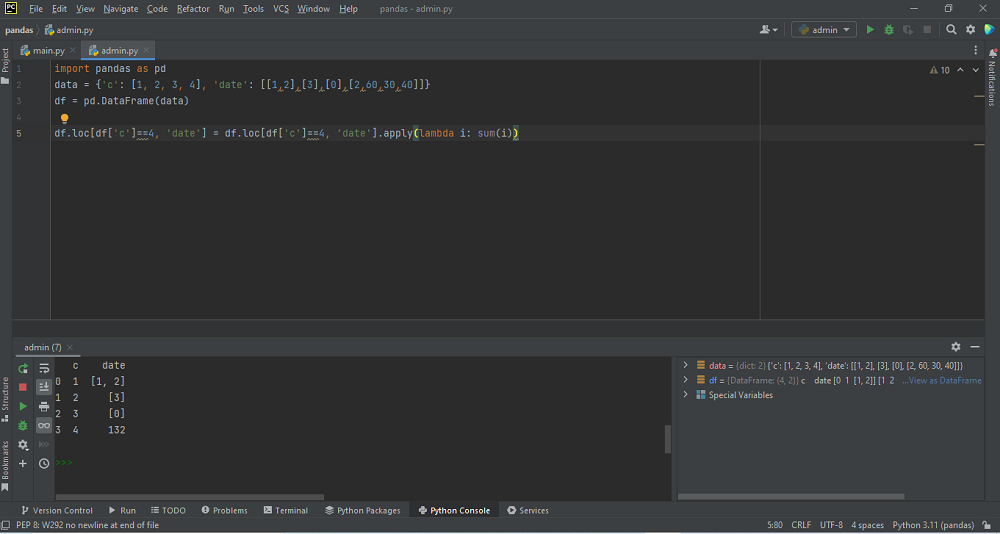# Convert Array to DataFrame using Pandas in Pythonby vsasikalabeUpdated: Feb 26, 2023Solution Kit

Pandas is a Python library. It is used for working with collections of data. This function is used for analyzing, cleaning, exploring, and manipulating data. A Data Frame is similar to a 2D array. Remember that the input array must be 2D. Otherwise, you will get a ValueError. If you pass a raw array, the index and column names start at 0 by default. In Python, a lambda function is a function. It can take any number of arguments and execute an expression. We can create this expression by using the lambda keyword.

Storing values in several dimensions are referred to as Multidimensional arrays. For example, an array in two dimensions is like a matrix, and an array in three dimensions can be a cube. In Python, using the NumPy library, multidimensional arrays are usually created. In this way, storing data can make it easier. And also to organize large amounts of data in a structure that is very easier to work with. A data frame is like an Excel sheet, i.e., a table of rows and columns. We can also create a Data Frame object from a dictionary of lists. The difference is that the key is the index, whereas the key is the column name in a Data Frame.

Parameters:

• The first parameter is data, and it is the only one necessary. The array is required to turn into a data frame.
• Index: It returns the index information of the Data frame. RangeIndex is used by default if we don’t use this parameter.
• columns: These are labels for the columns in a data frame. If we don’t use this argument RangeIndex (0, 1, 2,…, n) is used by default.

Here is an example of how to convert an Array to a Data frame using Pandas in Python:Preview of the output that you will get on running this code from your IDE.

### Code

In this solution we used pandas library of python.

### Instructions

Follow the steps carefully to get the output successfully:

2. Create new python file in your IDE.(Pycharm Preferable)
3. Copy the code using the "Copy" button above, and paste it in a Python file.
4. Run the file to generate the output.

I hope you found this useful. I have added the link to dependent libraries, version information in the following sections.

I found this code snippet by searching for "Convert Array to DataFrame using Pandas in Python"in kandi. You can try any such use case

### Environment Tested

I tested this solution in the following versions. Be mindful of changes when working with other versions.

1. The solution is created in Python 3.11.1 Version
2. The solution is tested on pandas 1.5.2 Version

Using this solution, we can Convert Array to DataFrame using Pandas in Python.This process also facilities an easy to use, hassle free method to create a hands-on working version of code in python.

### Dependent Library

pandasby pandas-dev

Python37360Version:v2.0.0rc1Flexible and powerful data analysis / manipulation library for Python, providing labeled data structures similar to R data.frame objects, statistical functions, and much more

Support
Quality
Security
Reuse

pandasby pandas-dev

Python37360Version:v2.0.0rc1License: Permissive (BSD-3-Clause)

Flexible and powerful data analysis / manipulation library for Python, providing labeled data structures similar to R data.frame objects, statistical functions, and much more
Support
Quality
Security